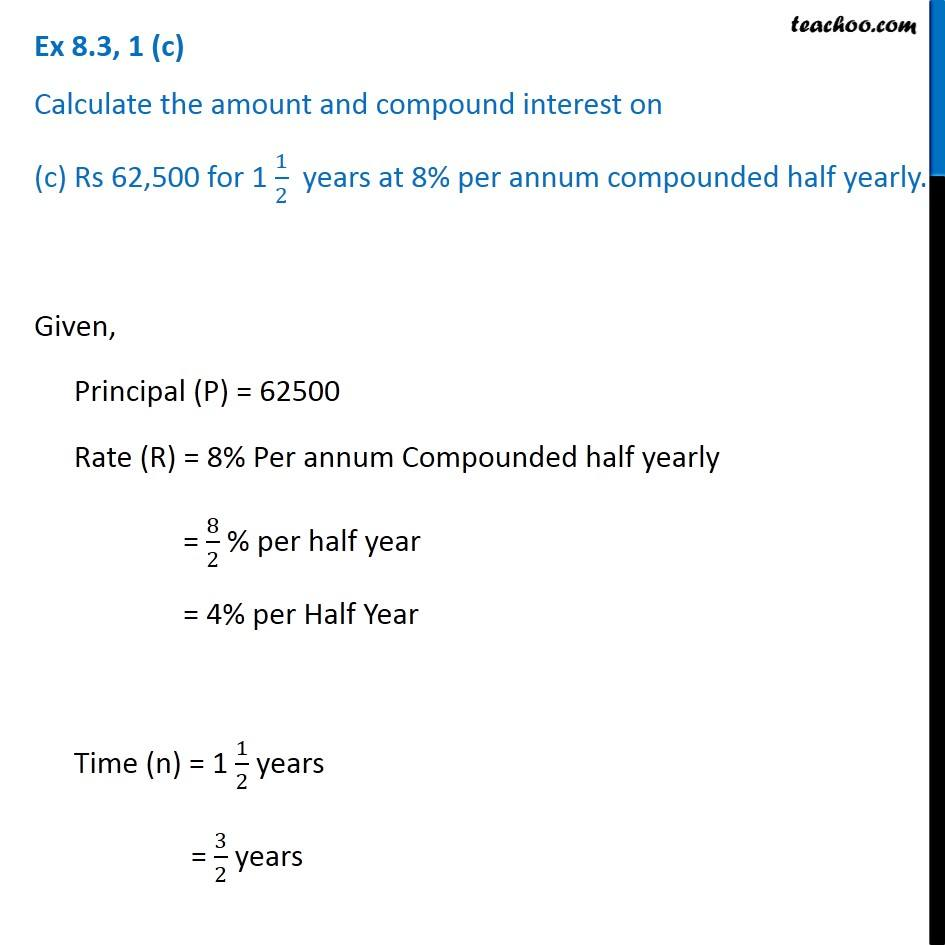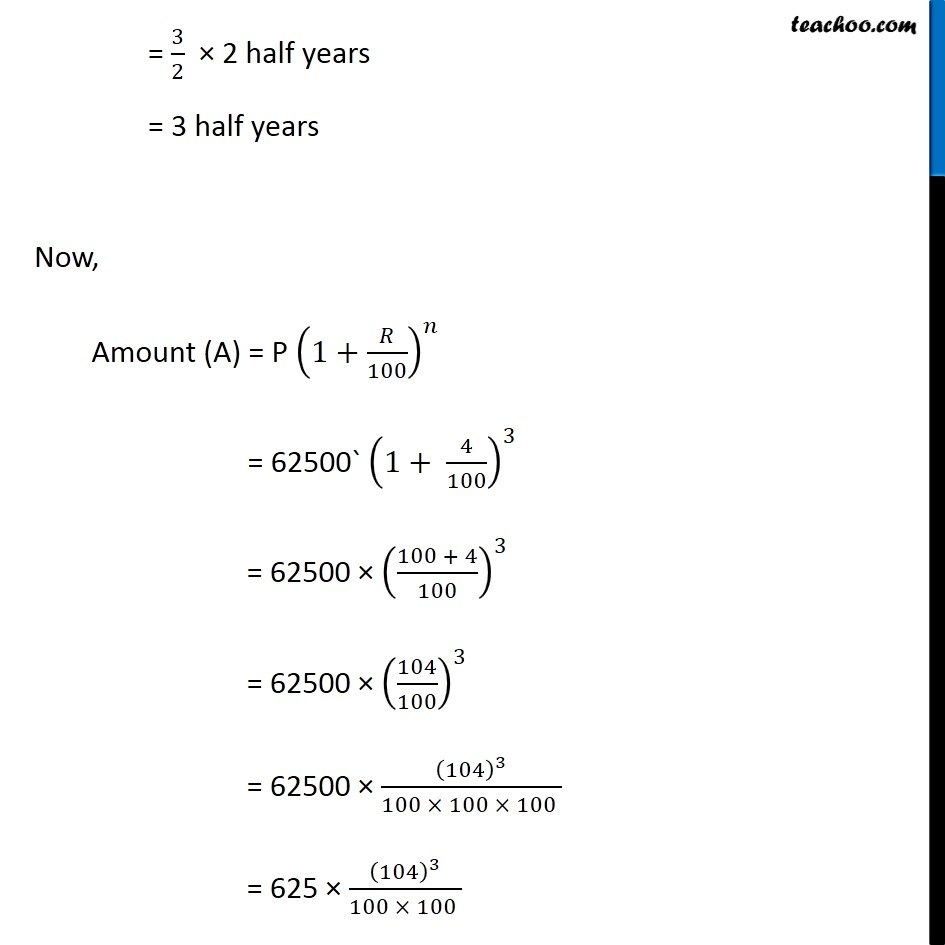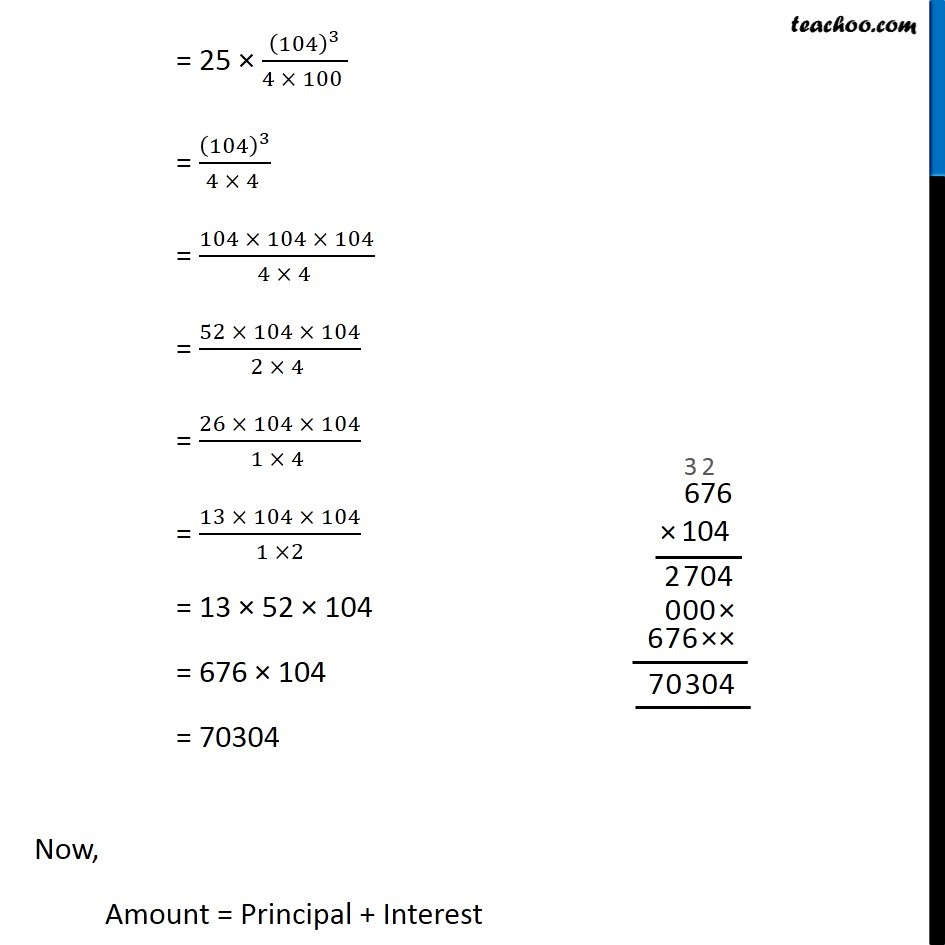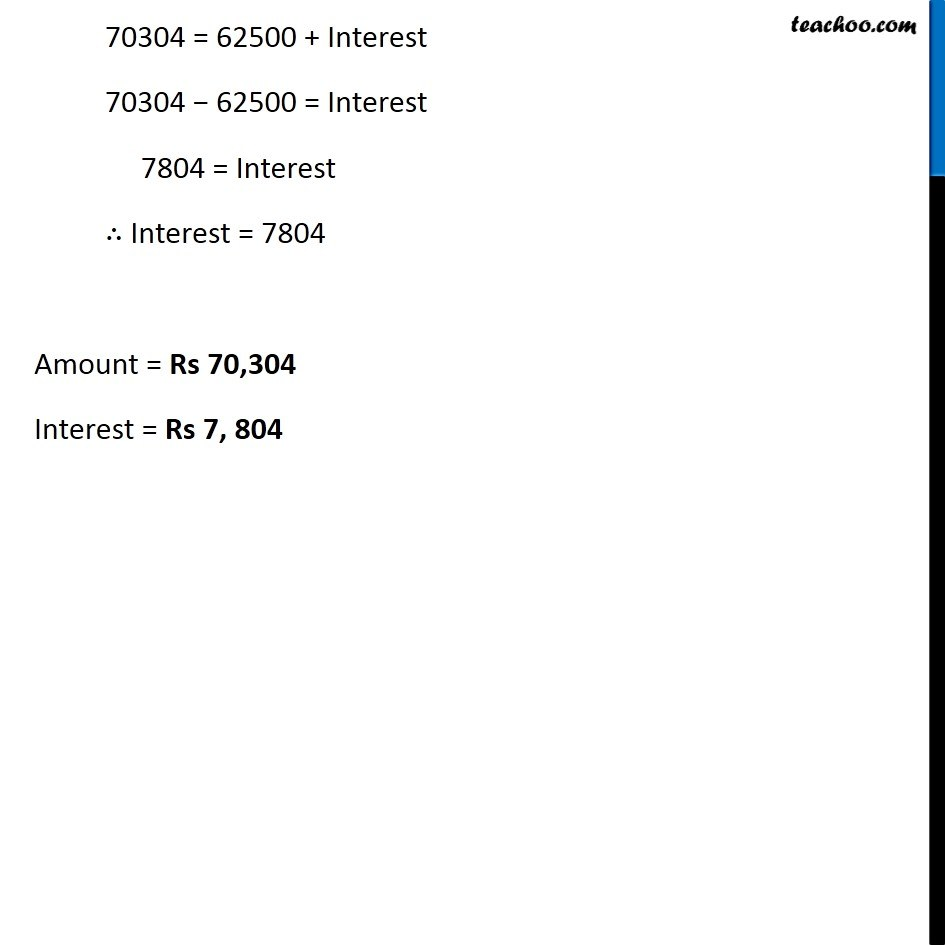Ex 8.3

Chapter 8 Class 8 Comparing Quantities
Serial order wiseGet live Maths 1-on-1 Classs - Class 6 to 12

### Transcript

Ex 8.3, 1 (c) Calculate the amount and compound interest on (c) Rs 62,500 for 1 1/2 years at 8% per annum compounded half yearly.Given, Principal (P) = 62500 Rate (R) = 8% Per annum Compounded half yearly = 8/2 % per half year = 4% per Half Year Time (n) = 1 1/2 years = 3/2 years = 3/2 × 2 half years = 3 half years Now, Amount (A) = P (1+𝑅/100)^𝑛 = 62500` (1+ 4/100)^3 = 62500 × ((100 + 4)/100)^3 = 62500 × (104/100)^3 = 62500 × (104)^3/(100 × 100 × 100 ) = 625 × (104)^3/(100 × 100 ) = 25 × (104)^3/(4 × 100 ) = (104)^3/(4 × 4 ) = (104 × 104 × 104)/(4 × 4 ) = (52 × 104 × 104)/(2 × 4 ) = (26 × 104 × 104)/(1 × 4 ) = (13 × 104 × 104)/(1 ×2) = 13 × 52 × 104 = 676 × 104 = 70304 Now, Amount = Principal + Interest 70304 = 62500 + Interest 70304 − 62500 = Interest 7804 = Interest ∴ Interest = 7804 Amount = Rs 70,304 Interest = Rs 7, 804